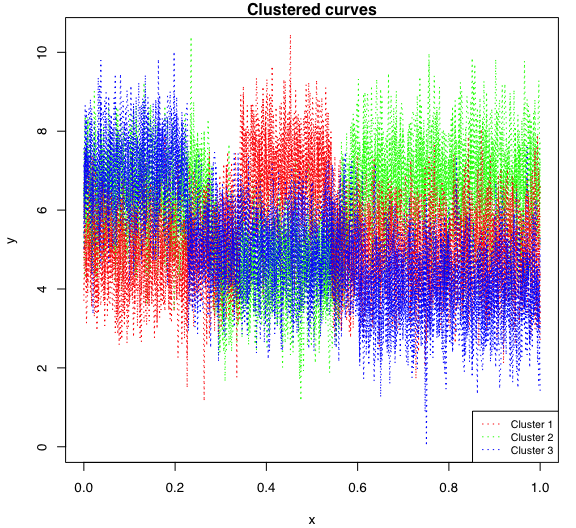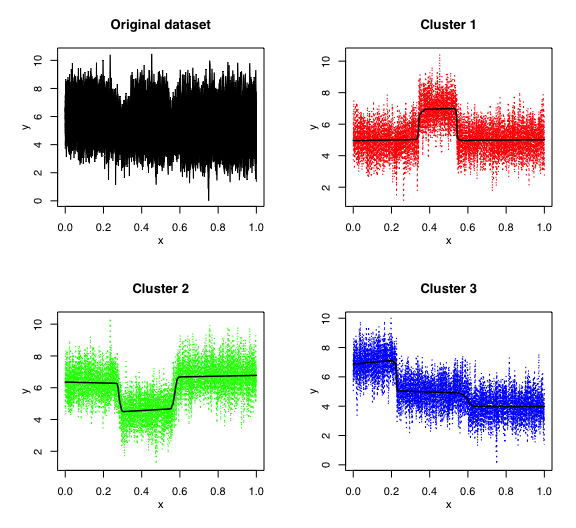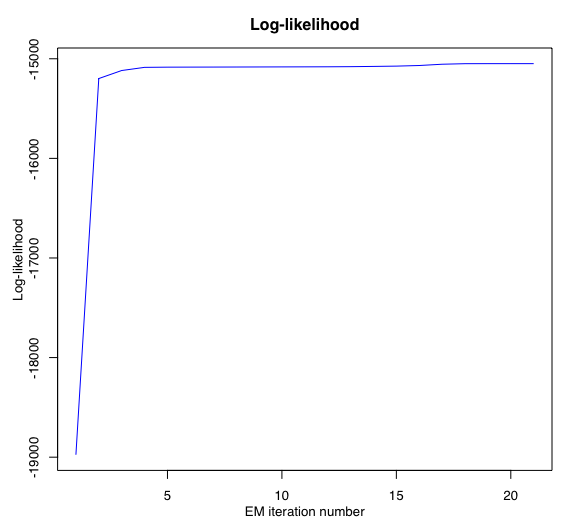# Introduction

mixHMMR: Simultaneous model-based clustering and segmentation of heterogeneous and dynamical functional data (curves/times series) with changes in regime by a mixture of gaussian regression models with hidden Markov processes, fitted by the EM/Baum-Welch algorithm.

It was written in R Markdown, using the knitr package for production.

See help(package="flamingos") for further details and references provided by citation("flamingos").

data("toydataset")
x <- toydataset$x Y <- t(toydataset[,2:ncol(toydataset)]) # Set up mixHMMR model parameters K <- 3 # Number of clusters R <- 3 # Number of regimes/states p <- 1 # Degree of the polynomial regression variance_type <- "heteroskedastic" # "heteroskedastic" or "homoskedastic" model # Set up EM parameters ordered_states <- TRUE n_tries <- 1 max_iter <- 1000 init_kmeans <- TRUE threshold <- 1e-6 verbose <- TRUE # Estimation mixhmmr <- emMixHMMR(X = x, Y = Y, K, R, p, variance_type, ordered_states, init_kmeans, n_tries, max_iter, threshold, verbose) ## EM - mixHMMR: Iteration: 1 || log-likelihood: -18975.6323298895 ## EM - mixHMMR: Iteration: 2 || log-likelihood: -15198.5811534058 ## EM - mixHMMR: Iteration: 3 || log-likelihood: -15118.0350455527 ## EM - mixHMMR: Iteration: 4 || log-likelihood: -15086.2933826057 ## EM - mixHMMR: Iteration: 5 || log-likelihood: -15084.2502053712 ## EM - mixHMMR: Iteration: 6 || log-likelihood: -15083.7770153797 ## EM - mixHMMR: Iteration: 7 || log-likelihood: -15083.3586992156 ## EM - mixHMMR: Iteration: 8 || log-likelihood: -15082.8291034608 ## EM - mixHMMR: Iteration: 9 || log-likelihood: -15082.2407744542 ## EM - mixHMMR: Iteration: 10 || log-likelihood: -15081.6808462523 ## EM - mixHMMR: Iteration: 11 || log-likelihood: -15081.175618676 ## EM - mixHMMR: Iteration: 12 || log-likelihood: -15080.5819574865 ## EM - mixHMMR: Iteration: 13 || log-likelihood: -15079.3118011276 ## EM - mixHMMR: Iteration: 14 || log-likelihood: -15076.8073408977 ## EM - mixHMMR: Iteration: 15 || log-likelihood: -15073.8399600893 ## EM - mixHMMR: Iteration: 16 || log-likelihood: -15067.6884092484 ## EM - mixHMMR: Iteration: 17 || log-likelihood: -15054.9127597414 ## EM - mixHMMR: Iteration: 18 || log-likelihood: -15049.4000307536 ## EM - mixHMMR: Iteration: 19 || log-likelihood: -15049.0221351022 ## EM - mixHMMR: Iteration: 20 || log-likelihood: -15048.997021329 ## EM - mixHMMR: Iteration: 21 || log-likelihood: -15048.9949507534 # Summary mixhmmr$summary()
## ------------------------
## Fitted mixHMMR model
## ------------------------
##
## MixHMMR model with K = 3 clusters and R = 3 regimes:
##
##  log-likelihood nu       AIC       BIC       ICL
##       -15048.99 50 -15098.99 -15134.02 -15134.02
##
## Clustering table (Number of curves in each clusters):
##
##  1  2  3
## 10 10 10
##
## Mixing probabilities (cluster weights):
##          1         2         3
##  0.3333333 0.3333333 0.3333333
##
##
## --------------------
## Cluster 1 (k = 1):
##
## Regression coefficients for each regime/segment r (r=1...R):
##
##     Beta(r = 1) Beta(r = 2) Beta(r = 3)
## 1     4.9512819   6.8393804   4.9076599
## X^1   0.2099508   0.2822775   0.1031626
##
## Variances:
##
##  Sigma2(r = 1) Sigma2(r = 2) Sigma2(r = 3)
##      0.9576192      1.045043      0.952047
##
## --------------------
## Cluster 2 (k = 2):
##
## Regression coefficients for each regime/segment r (r=1...R):
##
##     Beta(r = 1) Beta(r = 2) Beta(r = 3)
## 1     6.3552432   4.2868818   6.5327846
## X^1  -0.2865404   0.6907212   0.2429291
##
## Variances:
##
##  Sigma2(r = 1) Sigma2(r = 2) Sigma2(r = 3)
##      0.9587975     0.9481068       1.01388
##
## --------------------
## Cluster 3 (k = 3):
##
## Regression coefficients for each regime/segment r (r=1...R):
##
##     Beta(r = 1) Beta(r = 2) Beta(r = 3)
## 1      6.870328   5.1511267   3.9901300
## X^1    1.204150  -0.4601777  -0.0155753
##
## Variances:
##
##  Sigma2(r = 1) Sigma2(r = 2) Sigma2(r = 3)
##      0.9776399     0.9895623       0.96457

# Plots

mixhmmr\$plot()